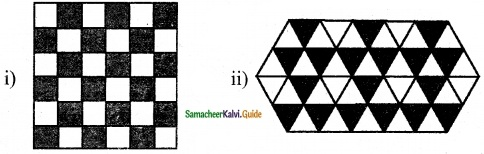Students can download Maths Chapter 4 Symmetry Ex 4.2 Questions and Answers, Notes, Samacheer Kalvi 6th Maths Guide Pdf helps you to revise the complete Tamilnadu State Board New Syllabus, helps students complete homework assignments and to score high marks in board exams.

## Tamilnadu Samacheer Kalvi 6th Maths Solutions Term 3 Chapter 4 Symmetry Ex 4.2

Miscellaneous Practice Problems

Question 1.
(i) A triangle which has no line of symmetry.
(ii) A triangle which has only one line of symmetry
(iii) A triangle which has three lines of symmetry.
Solution:
(i) Scalene triangle
(ii) Isosceles triangle
(iii) Equilateral triangleQuestion 2.
Find the alphabets in the box which have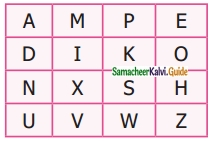(i) No line of symmetry
(ii) Rotational symmetry
(iii) Reflection symmetry
(iv) Reflection and rotational symmetry.
Solution:
i) The alphabets which have no line of symmetry are P, N, S, Z
ii) The alphabets which have Rotational symmetry are I, O, N, X, S, H, Z
iii) The alphabets which have reflection symmetry are A, M, E, D, I, K, O, X, H, U, V, W.
iv) The alphabets which has reflection and rotational symmetry are I, O, X, H.Question 3.
For the following pictures, find the number of lines of symmetry and also find the order of rotation.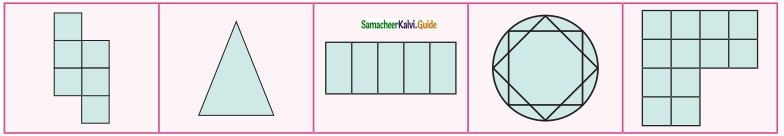Solution:
(i) 0, 2
(ii) 1, 0
(iii) 2, 2
(iv) 8, 8
(v) 1, 0Question 4.
The three-digit number 101 has rotational and reflection symmetry. Give five more examples of three-digit numbers that have both rotational and reflection symmetry
Solution:
The digits 0, 1, 8 have rotational and reflection symmetry.
∴ The three digits numbers 181, 111, 808, 818, 888 have both rotational and reflection symmetry.

Question 5.
Translate the given pattern and complete the design in a rectangular strips?Solution: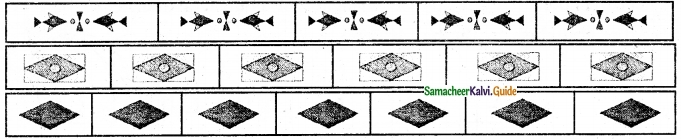Challenge Problems

Question 6.
Shade one square so that it possesses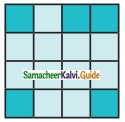(i) One line of symmetry
(ii) Rotational symmetry of order 2
Solution: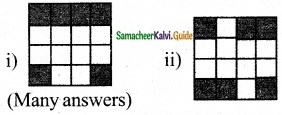Question 7.
Join six identical squares so that atleast one side of a square fits exactly with any other side of the square and has reflection symmetry (any three ways).
Solution: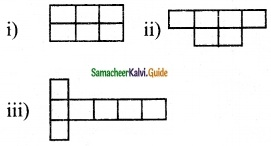Question 8.
Draw the following
(i) A figure which has reflection symmetry but no rotational symmetry.
(ii) A figure which has rotational symmetry but no reflection symmetry.
(iii) A figure which has both reflection and rotational symmetry.
Solution: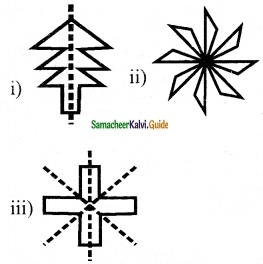Question 9.
Find the line of symmetry and the order of rotational symmetry’ of the given regular polygons and complete the following table and answer the questions given below.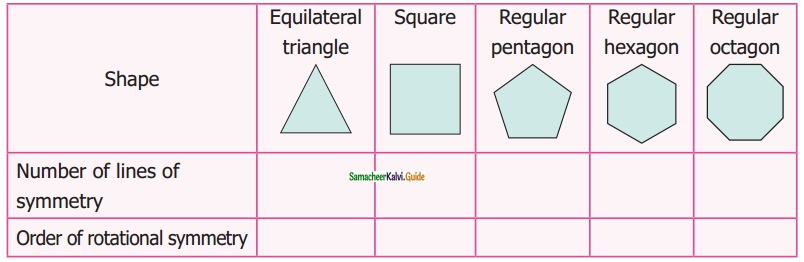i) A regular polygon of 10 sides will have ______ lines of symmetry.
ii) If a regular polygon has 10 lines of symmetry then its order of rotational symmetry is ______
iii) A regular polygon of ‘n’ sides has lines of symmetry and the order of rotational symmetry is ______
Solution:
(i) 10
(ii) 10
(iii) n, nQuestion 10.
Colour the boxes in such a way that it possesses translation symmetry.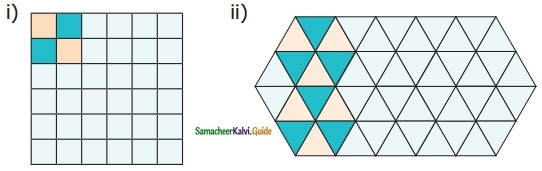Solution: